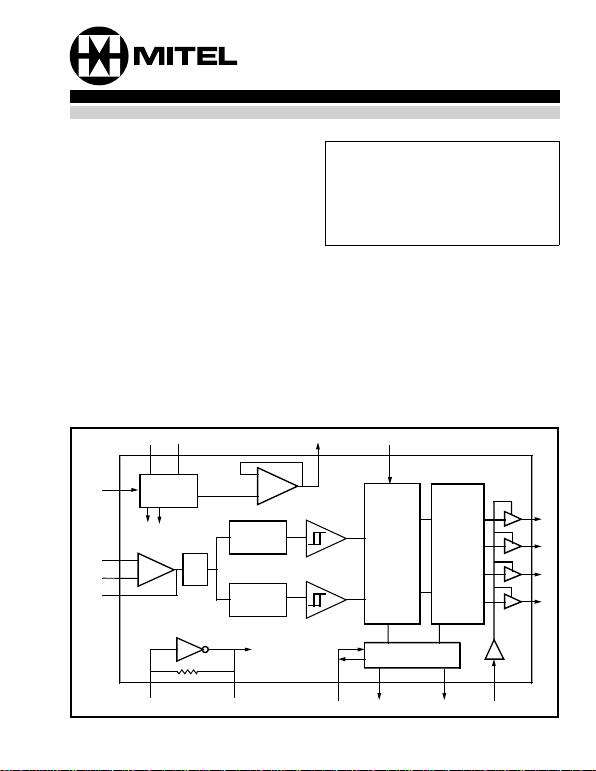4-11
MT8870D/MT8870D-1
Features
Comp let e DTMF R ecei ver
Low power co nsu mptio n
Intern al gai n sett ing a mplif ier
Cen tral office qua lity
Powe r-d own m ode
Inhi bi t mo de
Backward compatible with
MT8870C/MT8870C-1
Applications
Receiver system for British Telecom (B T) or
CEPT Spe c ( MT8870 D-1)
Paging systems
Credit card systems
Remo te con trol
Perso na l comput ers
Telep hone a nswe ring m ach ine
Description
The MT8870D/MT8870D-1 is a complete DTMF
receiver integrating both the bandsplit filter and
digital decoder functions. The filter section uses
switched capacitor techniques for high and low
group filters; the decoder uses digital counting
techniques to detect and decode all 16 DTMF tone-
pairs into a 4-bit code. External component count is
minimized by on chip provision of a differential input
am plifier, clock oscillator and latched three-state bus
interface.
Ordering Information
MT8870DE/DE-1 18 Pin Plastic DIP
MT8870DC/ DC-1 18 Pin Ceramic DIP
MT8870DS/DS-1 18 Pin SOIC
MT8 870DN/D N-1 20 Pin S SOP
MT8 870DT /DT-1 20 Pi n TSS OP
-40 °C to +85 °C
Figure 1 - Functional Block Diagram
PWDN
IN +
IN -
GS
OSC1 OSC2 St/GT ESt STD TOE
Q1
Q2
Q3
Q4
VDD VSS VRef INH
Bias
Circuit
Dial
Tone
Filter
High Group
Filter
Low Group
Filter
Digital
Detection
Algorithm
Code
Converter
and Latch
St
GT Steering
Logic
Chip
Power Chip
Bias
VRef
Buffer
Zero Crossing
Detectors
to all
Chip
Clocks
ISSUE 3 May1995
ISO2-CMOSMT8870D/MT8870D-1 ISO2-CMOS
4-12
Figure 2 - Pin Conne ctions
Pin Description
Pin #
Name Description
18 20
11 IN+ Non-Inverting Op-Amp (Input).
2 2 IN- Inverting Op-Amp (Input).
33 GS Gai n Sele ct. Gives access to output of front end different ial am plif ier for connection of
feedback resist or.
44 V
Ref Reference Voltage (Output ). Nominally VDD/2 is used to bias inputs at mid-rail (see Fig. 6
and Fig. 10 ).
55 INH Inhibit (Input). Logi c high inhibi ts the det ecti on of tones represe nti ng characters A, B, C
and D. This pin input is internally pulled down.
66PWDNPower Down (Input). Active hig h. Powers down the device and inhibit s the o scillato r. T his
pin input is inte rnally pulle d do wn.
78 OSC1Clock (Input).
89 OSC2Clock (Output). A 3.579545 MH z crystal connected betwe en pins OS C1 and OSC2
complet es the internal oscillat or cir cuit.
910 V
SS Ground (Input). 0V typical.
10 11 TOE Three S ta te Outpu t Ena bl e (Inp ut). Logic high e nables the outpu ts Q1-Q4. This pin is
pulled up inte rn ally.
11-
14 12-
15 Q1-Q4 Th ree S tate Data (Output). When enabl ed by TOE , provide t he co de corresponding to the
last valid ton e-pair received (see Table 1). When TOE is logic low, the data outputs are high
impedance.
15 17 StD Delayed Steering (Output).Present s a logic high when a received tone-pair has been
registered and the output latch updated; returns to logi c low when the voltage on St/GT falls
below VTSt.
16 18 ESt Early Steering (Output). Pre se nts a logic high once the digit al algorit hm has detect ed a
valid tone pair ( signal condition ). Any m ome ntary loss of signal condi tio n will cause ESt to
17 19 St/GT Steering Input/Guard time (Output) Bidirectional. A voltage greater than VTSt detected at
St causes the device to register the detected tone pair and update th e output latch . A
voltage less than V TSt f rees the device to accept a new tone pair. T he GT output acts to
reset the external ste ering time-const ant ; its state is a functi on of ESt and t he volta ge on St .
18 20 VDD Positive power supply (Input). +5V typical.
7,
16 NC No Connection.
1
2
3
4
5
6
7
8
910
18
17
16
15
14
13
12
11
IN+
IN-
GS
VRef
INH
PWDN
OSC1
OSC2
VSS
VDD
St/GT
ESt
StD
Q4
Q3
Q2
Q1
TOE
18 PIN CERDIP/P LAS TIC DIP/S OIC
1
2
3
4
5
6
7
8
9
10 11
12
20
19
18
17
16
15
14
13
IN+
IN-
GS
VRef
INH
PWDN
NC
OSC1
OSC2
VSS
20 PIN SSOP/TSSOP
VDD
St/GT
ESt
StD
Q4
Q3
Q2
Q1
TOE
NC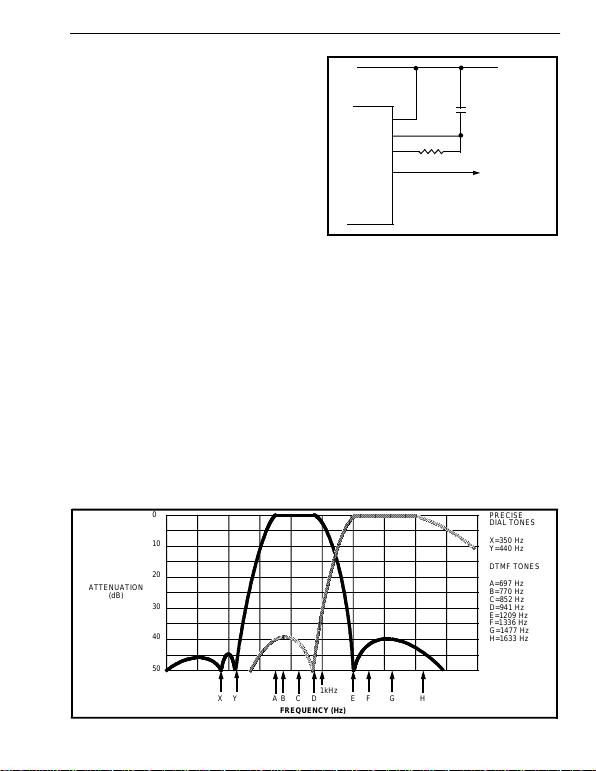ISO2-CMOS MT8870D/MT8870D-1
4-13
Functional Desc ription
The MT8870D/MT8870D-1 monolithic DTMF
receiver offers small size, low power consumption
and high performance. Its architecture consists of a
bandsplit filter section, which separates the high and
low group tones, followed by a digital counting
section which verifies the frequency and duration of
the received tones before passing the corresponding
code to the output bus.
Filter Section
Separation of the low-group and high group tones is
achieved by applying the DTMF signal to the inputs
of two sixth-order switched capacitor bandpass
filters, the bandwidths of whic h correspond to the low
and high group frequencies. The filter section also
incorporates notches at 350 and 440 Hz for
exceptional dial tone rejection (see Figure 3). Each
filter output is followed by a single order switched
capacitor filter section which smooths the signals
prior to limiting. Limiting is performed by high-gain
comparators which are provided with hysteresis to
prevent detection of unwanted low-level signals. The
outputs of the comparators provide full rail logic
swings at the frequencies of the incoming DTMF
signals.
D eco d er S ection
Following the filter section is a decoder employing
digital counting techniques to determine the
frequencies of the incoming tones and to verify that
they correspond to standard DTMF frequencies. A
complex averaging algorithm protects against tone
simul ation by ex traneous signal s such as voice whi le
Fi gure 4 - B asic Steer ing Circ u it
providing tolerance to small frequency deviations
and variations. This averaging algorithm has been
developed to ensure an optimum combination of
immunity to talk-off and tolerance to the presence of
interfering frequencies (third tones) and noise. When
the detector recognizes the presence of two valid
tones (this is referred to as the “signal condition” in
some industry specifications) the “Early Steering”
(ESt) output will go to an active state. Any
subsequent loss of signal condition will cause ESt to
assume a n in ac tive s ta te ( see “S te er in g Cir cu it”).
Steering Circuit
Before registration of a decoded tone pair, the
receiver checks for a valid signal duration (referred
to as character recognition condition). This check is
performed by an external RC time constant driven by
ESt. A logic high on ESt c auses vc (see Figure 4) to
rise as the capacitor discharges. Provided signal
VDD
C
vc
VDD
St/GT
ESt
StD
MT8870D/
MT8870D-1
R
tGTA=(RC)In(VDD/VTSt)
tGTP=(RC)In[VDD/(VDD-VTSt)]
Figure 3 - Filter Response
AA
AA
AA
AA
AAA
AAA
AA
AA
AA
AA
AA
AA
AA
AA
AA
AA
AA
AA
AA
AA
AA
AA
AAA
AAA
AAA
AAA
AA
AA
AA
AA
AA
AA
AA
AA
AA
AA
AA
AAA
AAA
AA
AA
AA
AA
AA
AA
AAA
AAA
AA
AA
AA
AA
AA
AA
AA
AA
AA
AA
AA
AA
AA
AA
AA
AAA
AAA
AA
AA
AA
AA
AA
AA
AA
AA
AA
AA
AA
AA
AA
AA
AA
AA
AA
AA
AA
AA
AA
AA
AAA
AAA
AA
AA
AA
AA
AA
AA
AA
AA
AAA
AAA
AA
AA
AA
AA
AA
AA
AAA
AAA
AA
AA
AA
AA
AA
AA
AA
AA
AA
AA
AA
AA
AA
AA
AA
AA
AA
AA
AA
AA
AA
AA
AA
AA
AA
AA
AA
AA
AA
AA
AA
AA
AA
AA
AA
AA
AA
AA
AA
AA
AA
AA
AA
AA
AA
AA
AA
AA
AA
AA
AA
AA
AA
AA
AA
AA
AA
AA
AA
AA
AA
AA
AA
AA
AA
AA
AA
AA
AA
AA
AA
AA
AA
AA
AA
AA
AA
AA
A
A
AA
AA
AA
AA
A
A
AA
AA
AA
AA
A
A
A
A
AA
AA
AA
AA
AA
AA
AA
AA
AA
AA
AA
AA
AA
AA
AA
AA
AA
AA
AA
AA
AA
AA
AA
AA
AA
AA
AA
AA
AA
AA
AA
AA
AA
AA
AA
AA
AA
AA
AAA
AAA
AA
AA
AA
AAA
AAA
AA
AA
AA
AA
AA
AA
AA
AA
AA
AA
AA
AA
AA
AA
AA
AA
AA
AA
AA
AA
AA
AA
AA
AAA
AAA
AA
AA
AA
AA
AA
AA
AA
AA
AA
AA
AA
AA
AA
AA
AAA
AAA
AA
AA
AA
AA
AA
AA
AA
AA
AA
AA
AA
AA
AA
AA
AA
AA
AA
AA
AA
AA
AA
AA
AA
AA
AA
AA
AA
AA
AA
AA
AA
AA
AA
AA
AAA
AAA
AA
AA
AA
AA
AA
AA
AAA
AAA
AAA
AA
AA
AA
AA
AA
AA
AA
AA
AA
AA
AA
AA
AA
AA
AA
AA
AA
AA
AAA
AAA
AA
AA
AA
AA
AA
AAA
AAA
AAA
AAA
AA
AA
AA
AA
AA
AA
AA
AA
AA
AA
AA
AA
AA
AA
AA
AA
AA
AA
AA
AA
AA
AAA
AAA
AAA
AAA
AAA
AAA
AA
AA
AAA
AAA
AA
AA
AA
AA
AA
AA
AA
AA
AA
AA
AA
AA
AA
AA
AA
AA
AA
AA
AA
AA
AAA
AA
AA
AA
AA
AA
AA
AAA
AAA
AAA
AA
AA
AA
AA
AA
AA
AA
AA
AA
AA
AA
AA
AA
AA
AA
AA
AA
AA
AA
AA
AA
AA
AA
AA
AA
AA
AA
AA
AA
AA
AA
AA
AA
AA
AAA
AAA
AAA
AAA
AAA
AA
AA
AAA
AAA
AA
AA
AA
AA
AA
AA
AA
AA
AA
AA
AA
AA
AA
AA
AA
AA
AA
AA
AA
AA
AA
AAA
AA
AA
AA
AA
AA
AA
AAA
AAA
AAA
AAA
AA
AA
AA
AA
AA
AA
AA
AA
AA
AA
AA
AA
AA
AA
AA
AA
AA
AA
AA
AA
AA
AA
AAA
AAA
AAA
AAA
AAA
AA
AAA
AAA
AA
AA
AA
AA
AA
AA
AA
AA
AA
AA
AA
AA
AA
AA
AA
AA
AA
AA
AA
AA
AAA
AA
AA
AA
AA
AA
AAA
AAA
AAA
AAA
AA
AA
AA
AA
AA
AA
AA
AA
AA
AA
AA
AA
AA
AA
AA
AA
AA
AA
AA
AA
AA
AA
AAA
AAA
AAA
AAA
AAA
AAA
AA
AA
AAA
AAA
AA
AA
AA
AA
AA
AA
AA
AA
AA
AA
AA
AA
AA
AA
AA
AA
AA
AAA
AA
AA
AA
AA
AA
AA
AAA
AAA
AAA
AAA
AA
AA
AA
AA
AA
AA
AA
AA
AA
AA
AA
AA
AA
AA
AA
AA
AA
AA
AAA
AAA
AA
AA
AA
AA
AA
AA
AAA
AAA
AAA
AA
AA
AA
AA
AA
AA
AA
AA
AA
AA
AA
AA
AA
AA
AA
AA
AA
AA
AA
AA
AA
AA
AA
AA
AAA
AAA
AA
AA
AAA
AAA
AAA
AAA
AAA
AAA
AA
AA
AA
AA
AA
AA
AA
AA
AA
AA
AA
AA
AA
AA
AA
AA
AA
AA
AA
AA
AA
AA
AA
AA
AA
AA
AA
AA
AA
AA
AA
AA
AA
AA
AA
AA
AA
AA
AA
AA
AA
AA
AA
AAA
AAA
AA
AA
AA
AAA
AAA
AA
AA
AAA
AAA
AAA
AAA
AA
AA
AA
AA
AA
AA
AAA
AAA
AA
AA
AA
AA
AAA
AAA
AA
AA
AA
AA
AA
AA
AA
AA
AA
AA
AA
AA
AA
AA
AA
AA
AA
AA
AA
AA
AA
AA
AA
AA
AA
AA
AA
AA
AA
AA
AA
AA
AA
AA
AA
AA
AA
AA
AAA
AAA
AA
AA
AA
AA
AA
AA
AA
AA
AA
AA
AA
AA
A
A
AA
AA
AA
AA
AA
AA
AAA
AAA
AA
AA
AA
AA
AA
AA
AA
AA
AA
AA
AA
AA
AA
AA
A
A
AA
AA
AA
AA
AA
AA
AA
AA
AA
AA
AA
AA
AA
AA
AA
AA
AA
AA
A
AAA
AAAA
AAAA
AAAA
AAAA
AAAA
AAAA
AAAA
AA
A
AAA
AA
AA
AA
AA
A
A
AA
AA
AA
AA
AA
AA
AA
AA
A
A
AA
AA
AA
AA
AA
AA
AA
AA
AA
AA
AA
AA
AA
AA
AA
AA
AA
AA
AA
AA
AA
AA
AA
AA
AA
AA
AA
AA
AA
AA
AA
AA
AA
AA
AA
AA
AA
AA
AAA
AAA
AA
AA
AA
AA
AA
AA
AA
AA
AA
AA
AA
AA
AA
AA
AA
AA
AA
AA
AA
AA
AA
AA
AA
AA
AA
AA
AA
AA
AA
AA
AA
AA
AA
AA
AA
AA
AA
AA
AA
AA
AA
AA
AA
AA
AA
AA
AA
AA
AA
AA
AA
AA
AA
AA
AA
AA
AA
AA
AA
AA
AA
AA
AA
AA
AA
AA
AA
AA
AA
AA
AA
AAA
AAA
AA
AA
AA
AA
AA
AA
AA
AA
AA
AA
AAA
AAA
AAA
AAA
AAA
AA
AA
AA
AA
AA
AA
AA
AA
AAA
AAA
AAA
AAA
AA
AA
AA
AA
AA
AA
AA
AA
AAA
AAA
AAA
AAA
AA
AA
AA
AA
AA
AA
AA
AA
AA
AA
AA
AA
AA
AA
AA
AA
AA
AA
AA
AA
AA
AA
AA
AA
AA
AA
AA
AA
AA
AA
AA
AA
AA
AA
AA
AA
AA
AA
AAA
AAA
AAA
AAA
AA
AA
AA
AA
AA
AA
AA
AA
AA
AA
AA
AA
AA
AA
AA
AA
AA
AA
AA
AA
AA
0
10
20
30
40
50
ATTENUATION
(dB)
XY ABCD
1kHz EF G H
PRECISE
DIAL TONES
X= 350 H z
Y= 440 H z
DTMF TONE S
A= 697 H z
B= 770 H z
C=85 2 Hz
D=94 1 Hz
E=1209 Hz
F=1336 Hz
G=1477 Hz
H=1633 Hz
FREQUENCY (Hz)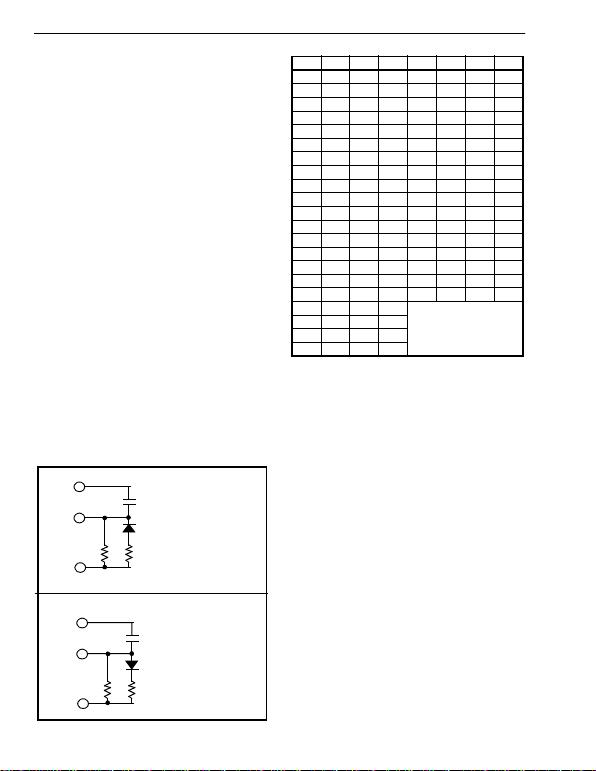MT8870D/MT8870D-1 ISO2-CMOS
4-14
condition is maintained (ESt remains high) for the
validation period (tGTP), vc reaches the threshold
(VTSt) of the steering logic to register the tone pair,
latching its corresponding 4-bit code (see Table 1)
into the output latch. At this point the GT output is
activated and drives vc to VDD. GT continues to drive
high as long as ESt remains high. Finally, after a
short delay to allow the output latch to settle, the
delayed steering output flag (StD) goes high,
signalling that a received tone pair has been
registered. The contents of the output latch are made
available on the 4-bit output bus by raising the three
state control input (TOE) to a logic high. The
steering circuit works in reverse to validate the
interdigit pause between signals. Thus, as well as
rejecting signals too short t o be considered valid, the
receiver will tolerate signal interruptions (dropout)
too short to be considered a valid pause. This facilit y,
together with the capability of selecting the steering
time constants externally, allows the designer to
tailor performance to meet a wide variety of system
requirements.
In many situations not requiring selection of tone
duration and interdigital pause, the simple steering
circuit shown in Figure 4 is applicable. Component
values are chosen according to the formula:
t
REC
=t
DP
+t
GTP
t
ID
=t
DA
+t
GTA
The value of tDP is a device parameter (see Figure
11) and tREC is the minimum signal duration to be
recognized by the receiver. A value for C of 0.1 µF is
Figure 5 - Gua rd Time Adjus tment
VDD
St/GT
ESt
C1
R1R2
a) decreasing tGTP; (tGTP<tGTA)
tGTP=(RPC1)In[VDD/(VDD-VTSt)]
tGTA=(R1C1)In(VDD/VTSt)
RP=(R1R2)/(R1+R2)
VDD
St/GT
ESt
C1
R1R2
tGTP=(R1C1)In[VDD/(VDD-VTSt)]
tGTA=(RPC1)In(VDD/VTSt)
RP=(R1R2)/(R1+R2)
b) decreasing tGTA; (tGTP>tGTA)
Table 1. Functional Decode Table
L=LOGIC LOW, H= LOGIC HIGH, Z=HIGH IMPEDANCE
X = DON‘T CA RE
recommended for most applications, leaving R to be
selected by the designer.
Different steering arrangements may be used to
select independently the guard times for tone
present (tGTP) and tone absent (tGTA). This may be
necessary to meet system specificat ions which place
both accept and reject limits on both tone duration
and interdigital pause. Guard time adjustment also
allows the designer to tailor system parameters
such as talk off and noise immunity. Increasing tREC
improves talk-off performance since it reduces the
probability that tones simulated by speech will
maintain signal condition long enough to be
registered. Alternatively, a relatively short tREC with
a long tDO would be appropriate for extremely noisy
environments where fast acquisition time and
immunity to tone drop-outs are required. Design
information for guard time adjustment is shown in
Figure 5.
Digit TOE INH ESt Q4Q3Q2Q1
ANYLXHZZZZ
1HXH0001
2HXH0010
3HXH0011
4HXH0100
5HXH0101
6HXH0110
7HXH0111
8HXH1000
9HXH1001
0HXH1010
*HXH1011
#HXH1100
AHLH1101
BHLH1110
CHLH1111
DHLH0000
AHHL
undetected, the output code
will remain the same as the
previous detec ted code
BHHL
CHHL
DHHL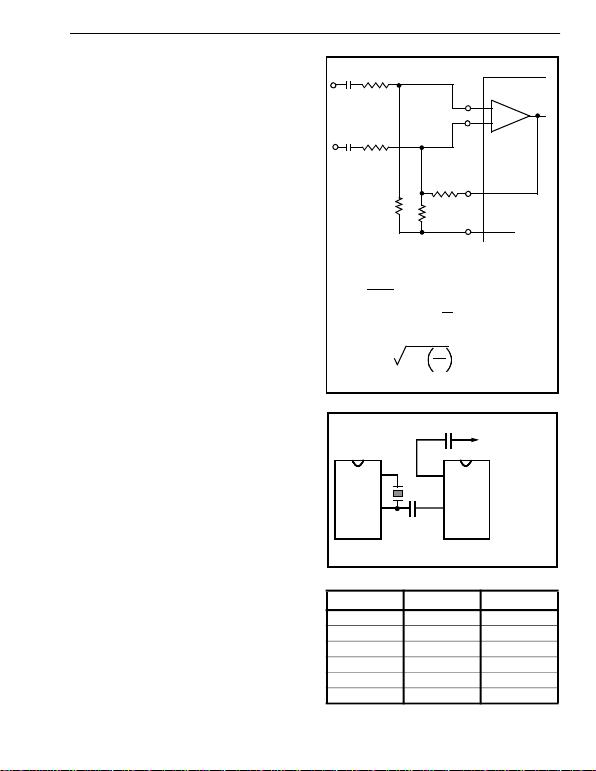ISO2-CMOS MT8870D/MT8870D-1
4-15
Power-down and Inhibit Mode
A logic high applied to pin 6 (PWDN) will power down
the device to minimize the power consumption in a
standby mode. It stops the oscillator and the
functions of the filters.
Inhibit mode is enabled by a logic high input to the
pin 5 (INH). It inhibits the detection of tones
representing characters A, B, C, and D. The output
code will remain the same as the previous detected
code (see Table 1).
Differential Input Configuration
The input arrangement of the MT8870D/MT8870D-1
provides a differential-input operational amplifier as
well as a bias s ource (VRef) w hich is u se d to b ias th e
inputs at mid-rail. Provision is made for connection of
a feedback resistor to the op-amp output (GS) for
adjustment of gain. In a single-ended configuration,
the input pins are connected as shown in Figure 10
with the op-amp connected for unity gain and VRef
biasing the input at 1/2VDD. Figure 6 shows the
differential configuration, which permits the
adjustment of gain with the feedback resistor R5.
Crystal Os cillator
The internal clock circuit is completed with the
addition of an external 3.579545 MHz crystal and is
normally connected as shown in Figure 10 (Single-
Ended Input Configuration). However, it is possible
to configure several MT8870D/MT8870D-1 devices
employing only a single oscillator crystal. The
oscillator output of the first device in the chain is
coupled through a 30 pF capacitor to the oscillator
input (OSC1) of the next device. Subsequent devic e s
are connected in a similar fashion. Refer to Figure 7
for details. The problems associated with
arrangement shown, i.e., precision balancing
capacitors are not required.
Figure 6 - Differential Input Configura tion
Figure 7 - Oscillator Connection
Table 2. Recom mended Resonator Specificati ons
Note : Qm= qu al ity f ac to r of R L C m od el , i .e. , 1 /2ΠƒR1C1.
Parameter Unit Resonator
R1 Ohms 10.752
L1 mH .432
C1 pF 4.984
C0 pF 37.915
Qm - 896.37
f % ±0.2%
C1R1
C2R4
R3
IN+
IN-
+
-
R5GS
R2VRef
MT8870D/
MT8870D-1
Differential Input Amplifier
C1=C2=10 nF
R1=R4=R5=100 k
R2=60k, R3=37.5 kAll resistors are ±1% tolerance.
All capacitors are ±5% tolerance.
R3=R2R5
R2+R5
VOLTAGE GAIN (Av diff)= R5
R1
INPUT IMPEDANCE
(ZINDIFF) = 2 R12+1
ωc
2
OSC1
OSC2
OSC2
OSC1
C
X-tal
C
To OSC1 of next
MT8870D/MT8870D-1
C=30 pF
X-tal=3.579545 MHz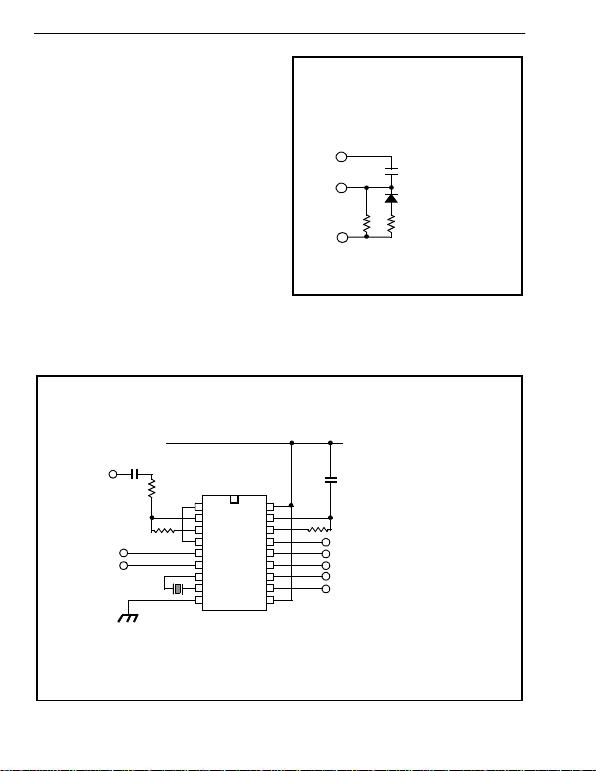MT8870D/MT8870D-1 ISO2-CMOS
4-16
Applications
RECEIVER SYSTEM FOR BRIT IS H TEL ECO M
SPEC POR 1151
The circuit shown in Fig. 9 illustrates the use of
MT8870D-1 device in a typical receiver system. BT
Spec defines the input signals less than -34 dBm as
the non-operate level. This condition can be
attained by choosing a suitable values of R1 and R2
to provide 3 dB attenuation, such that -34 dBm input
signal will correspond to -37 dBm at the gain setting
pin GS of MT8870D-1. As shown in the diagram, the
component values of R3 and C2 are the guard time
requirements when the total component tolerance is
6%. For better performance, it is recommended to
use the non-symmetric guard time circuit in Fig. 8.
Figure 8 - Non-Symmetric Guard Time Circuit
tGTP=(RPC1)In[VDD/(VDD-VTSt)]
tGTA=(R1C1)In(VDD/VTSt)
RP=(R1R2)/(R1+R2)
VDD
St/GT
ESt
C1
R2
R1Notes:
R1=368K Ω ± 1%
R2=2.2M Ω ± 1%
C1=100nF ± 5%
Figure 9 - Single-Ended Input Configuration for BT or CEPT Spec
IN+
IN-
GS
VRef
INH
PWDN
OSC 1
OSC 2
VSS TOE
VDD
St/GT
ESt
StD
Q4
Q3
Q2
Q1
DTMF
Input
C1
R1
R2
X1
VDD
C2
R3
MT8870D-1
NOTES:
R1 = 102KΩ ± 1%
R2 = 71.5KΩ ± 1%
R3 = 390KΩ ±1 %
C1,C2 = 100 nF ± 5%
X1 = 3.579545 MHz ± 0.1%
VDD = 5.0V ± 5%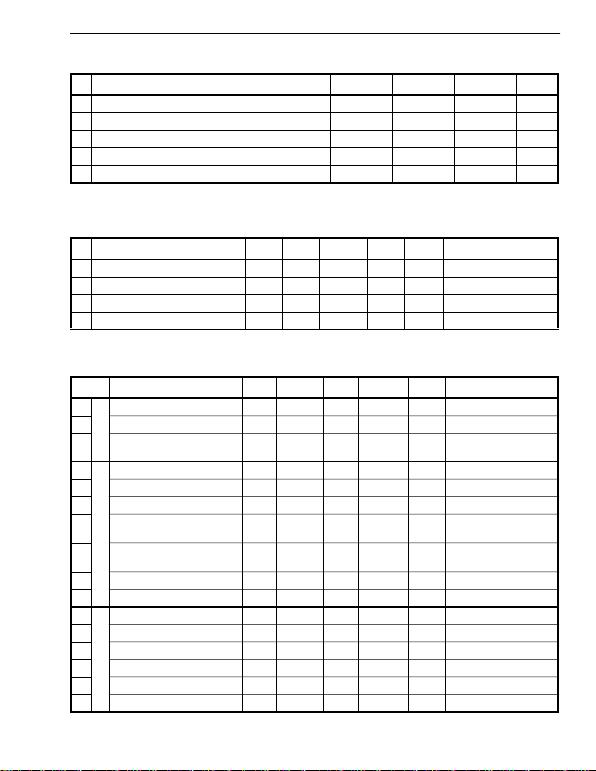ISO2-CMOS MT8870D/MT8870D-1
4-17
Exceeding these values may cause permanent damage. Functional operation under these conditions is not implied.
Dera te ab ove 75 ° C at 16 m W / ° C . All le ads s old ered to boa r d.
Typ ical figu res are at 25°C and are for desi gn aid only: not gua rante ed and not subj ect to producti on te stin g.
Typ ical figu res are at 25°C and are for desi gn aid only: not gua rante ed and not subj ect to producti on te stin g.
Ab solu te Maximum Ratings
Parameter Symbol Min Max Units
1 DC Power S upply Vo ltag e VDD 7V
2 Voltage on any pin VIVSS-0.3 VDD+0.3 V
3 Curre nt at any pin (other than suppl y) II10 mA
4 Storage t emp erature TSTG -65 +150 °C
5 Package pow er dissipation PD500 mW
Recommended Operating Conditions - Volt ag es are with respect to groun d (VSS) un less otherwise stated.
Parameter Sym Min TypMax Units Test Conditions
1 D C Power S upply Volta ge VDD 4.75 5.0 5.25 V
2 O perating Tem perat ure TO-40 +85 °C
3 C rystal/Clock Frequency fc 3.579545 MHz
4 C rystal/ Clock Freq.Tolerance fc ±0.1 %
DC Electrical Characteristics - VDD=5.0 5%, VSS=0V, -4 C TO +85°C, unless otherwise stated.
Characteristics Sym Min TypMax Units Test Conditions
1S
U
P
P
L
Y
Standby supply current IDDQ 10 25 µA PWDN=VDD
2 Operating supply current IDD 3.0 9.0 mA
3 Power consump tion PO15 mW fc=3.579545 MHz
4
I
N
P
U
T
S
High level input VIH 3.5 V VDD=5.0V
5 Low level input volta ge VIL 1.5 V VDD=5.0V
6 Input leakage current IIH/IIL 0.1 µAV
IN=VSS or VDD
7 Pull up (source) current ISO 7.5 20 µA TOE (p in 10)=0,
VDD=5.0V
8 Pull down ( sink ) current ISI 15 45 µA INH=5.0V, PWDN=5.0V,
VDD=5.0V
9 Input impedance (IN+, IN-) RIN 10 M@ 1 kHz
1 0 Steering thresho ld voltage VTSt 2.2 2.4 2.5 V VDD = 5.0V
11
O
U
T
P
U
T
S
Low level output voltag e VOL VSS+0.03 V No load
12 High level output voltage VOH VDD-0.03 V No load
1 3 Ou tput low (si nk ) current IOL 1.0 2.5 mA VOUT=0.4 V
14 Output high (source) current IOH 0.4 0.8 mA VOUT=4.6 V
15 VRef output voltage VRef 2.3 2.5 2.7 V No load, VDD = 5.0V
16 VRef output resistance ROR 1k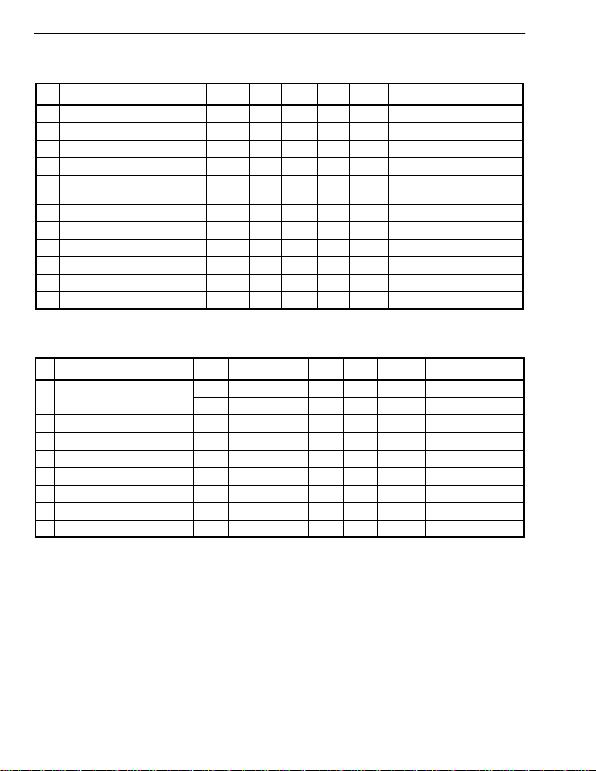MT8870D/MT8870D-1 ISO2-CMOS
4-18
Typ ical figu res are a t 25 °C an d are for desig n aid only: not gu arante ed and not subject to produ ct ion testin g.
*NOTES
1. dB m= decib el s ab ove or be low a ref erenc e pow er of 1 mW into a 60 0 ohm lo ad .
2. Digit sequence consists of all DTMF tones.
3. Tone du r a tio n= 4 0 m s , ton e pa us e= 4 0 m s .
4. Si gn al c on ditio n c o ns is ts o f n om in al D TM F fr e qu en ci es .
5. Bo th to nes in co m po si t e si gn al h av e an equa l am pl itud e.
6. Tone pa ir is d ev ia ted b y ±1 .5 %± 2 H z .
7. Ba nd w id th limi te d ( 3 k H z ) G a us si an n oi se .
8. The precise dial tone frequencies are (350 Hz and 440 Hz) ± 2 %.
9. Fo r a n err o r rate of bette r t ha n 1 i n 1 0, 00 0.
10. R e fe r en ced to lo w es t l ev e l f r eq ue nc y co m po ne nt in D TM F si gn al .
11. R e fe ren c ed to th e mi nimu m v ali d ac ce p t le v el .
12. Guaranteed by design and characterization.
Operating Characteristics - VDD=5.0V±5%, VSS=0 V, -40° C TO +85°C ,unless otherwise stated.
Gain Settin g Amplifier
Characteristics Sym Min TypMa x Un its Test Co nditions
1 I nput leakage current IIN 100 nA VSS VIN VDD
2 I nput resi stance RIN 10 M
3 I nput offset volta ge VOS 25 mV
4 P owe r supply rejection PSRR 50 dB 1 kHz
5 C o mmon m ode rejecti on CM R R 40 dB 0.75 V VIN 4.25 V biased
at VRef =2.5 V
6 DC open loop voltage gain AVOL 32 dB
7 U n ity gain bandwidt h fC0.30 MHz
8 O ut put volt age swing VO4.0 Vpp Load 100 k to VSS @ GS
9 Maxim um capacitive load (GS ) CL100 pF
1 0 R e sistive load (GS) RL50 k
11 Co mmon m ode range VCM 2.5 Vpp No Load
MT8870D AC Electrical Characteristics -V
DD=5.0V ±5%, VSS=0V, -40°C TO +8C , using Test Circuit shown in
Figure 10.
Characteristics Sym Min TypMax Units Notes*
1Valid input signal levels (each
tone of composit e signal) -29 +1 dBm 1,2,3,5,6,9
27.5 869 mVRMS 1,2,3,5,6,9
2 Neg ative twist accept 8 dB 2,3,6,9,12
3 P osit ive twist accept 8 dB 2, 3,6, 9, 12
4 F requency d eviat ion ac cept ±1. 5% ± 2 Hz 2,3,5, 9
5 Frequency deviat ion reject ±3.5% 2,3,5,9
6 T hird tone tolerance -16 dB 2,3,4, 5, 9,10
7 Noise tolerance -12 dB 2,3,4,5,7,9,10
8 Dial tone tolerance +22 dB 2,3,4,5,8,9,11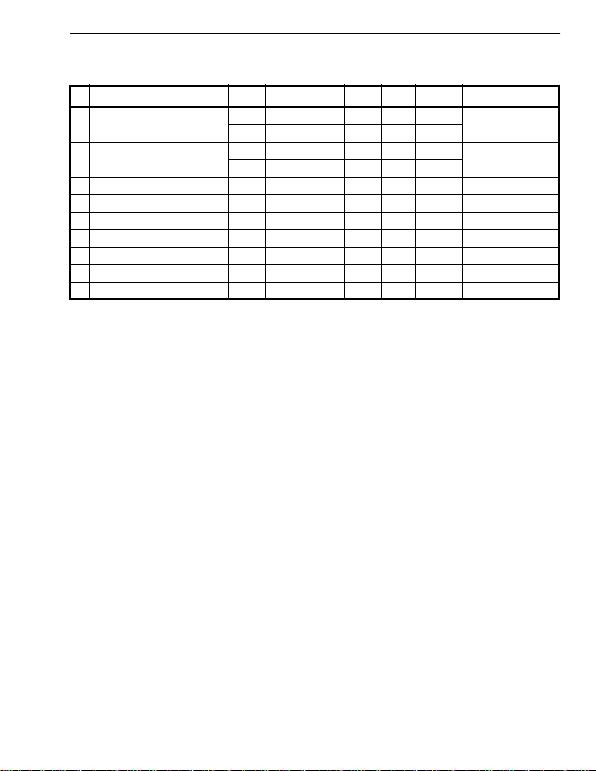ISO2-CMOS MT8870D/MT8870D-1
4-19
Typ ical figu res are at 25 °C and are fo r de sig n a id only: no t gu ara nteed and not subject to produ ct ion testin g.
*NOTES
1. dBm= decibels above or below a reference power of 1 mW into a 600 ohm load.
2. D ig it se qu en c e c o ns is ts of a ll D TM F t on es .
3. Tone du r at ion = 40 m s, ton e p au s e= 40 m s .
4. S ig na l co nd iti on c on s is ts of no m in al D TM F freque n ci es .
5. B o th ton es i n c omp os i t e si gn al ha v e a n eq ua l am pl itude .
6. Tone pa ir i s de vi at ed by ±1 .5 2 H z .
7. B a nd w id th lim i t ed (3 k H z ) G au s si an no is e .
8. The p r e ci se d ia l to ne fr eq ue nc ie s ar e (3 50 H z a nd 44 0 H z) ± 2 %.
9. For an e rr o r rate of bette r th an 1 in 1 0,0 00 .
10. R e fe r en ced to lo w es t l ev e l f r eq ue nc y co m po ne nt in D TM F si gn al .
11. R e fe ren c ed to th e mi nimu m v ali d ac ce p t le v el .
12. Referenced to Fig. 10 input DTMF tone level at -25dBm (-28dBm at GS Pin) interference frequency range between 480-3400Hz.
13. Guaranteed by design and characterization.
MT8870D-1 AC Electrical Characteristics -V
DD=5.0V±5%, VSS=0V, -40°C TO +85°C , using Test Circuit shown in
Figure 10.
Characteristics Sym Min TypMax Units Notes*
1Valid input signal levels (each
tone of composit e signal) -31 +1 dBm T ested at VDD=5.0V
1,2,3,5,6,9
21.8 869 mVRMS
2 Input Signal Level Reject -37 dBm T ested at VDD=5.0V
1,2,3,5,6,9
10.9 mVRMS
3 Negative twist accept 8 dB 2,3,6,9,13
4 Posit ive twi st accep t 8 dB 2, 3,6, 9, 13
5 Frequency deviat ion accept ±1. 5%± 2 Hz 2,3,5,9
6 Frequency deviat ion reject ±3.5% 2,3,5, 9
7 Third zone tolerance -18.5 dB 2, 3,4,5,9,12
8 Noise tolerance -12 dB 2,3,4,5,7,9,10
9 Dial tone tolerance +2 2 dB 2, 3,4, 5, 8,9 ,11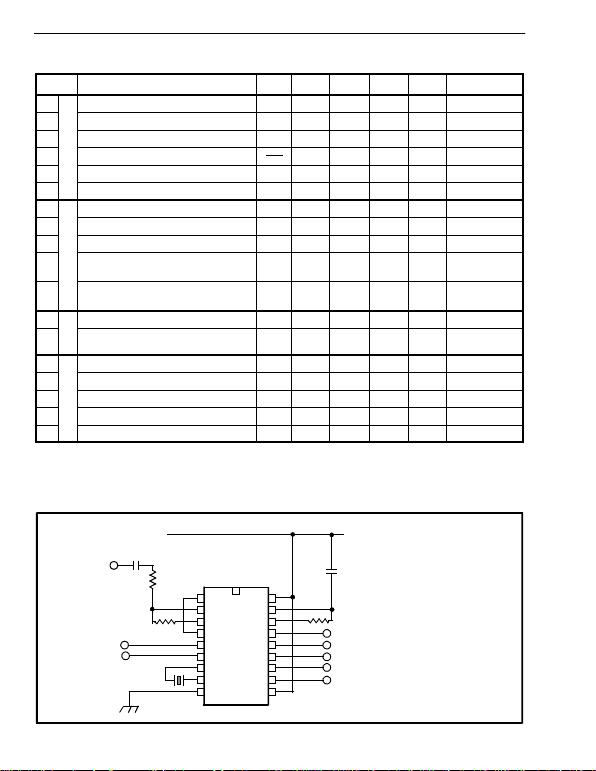MT8870D/MT8870D-1 ISO2-CMOS
4-20
Typ ical figu res are a t 25°C and a re f or desi gn aid only: no t gua ran teed and no t subject to prod ucti on testin g.
*NOTES:
1. Us ed fo r gua r d-t im e c a lc ul ati on p ur p os e s o nl y.
2. Thes e, us e r a dju s tab le p ar a m ete rs , are no t dev ic e sp ec if ic ations . The adj us table s ettin gs of the se m inim um s a nd m ax im u ms
are recommendations based upon network requirements.
3. Wi th va li d ton e pr e se nt at in put, tPU eq ua ls t im e fr om P D WN g oi ng l ow u nt il E St goin g hig h.
Figure 10 - Single-Ended Input Configuration
AC Electrical Characteristics - VDD=5.0V±5%, VSS=0V, -40°C To +85°C , using Test Circuit shown in Figure 10.
Characteristics Sym Min TypMax Units Conditions
1
T
I
M
I
N
G
Tone present det ect tim e tDP 5 11 14 ms Note 1
2 Tone absent de tect t im e tDA 0.5 4 8.5 ms Note 1
3 Tone duration acce pt tREC 40 ms Note 2
4 Tone durat ion reject tREC 20 ms Note 2
5 Int erdigit pause accept tID 40 ms Note 2
6 Int erdigi t pause reject tDO 20 ms Note 2
7
O
U
T
P
U
T
S
Propa gati on delay (St to Q) tPQ 811µsTOE=V
DD
8 Propa gati on delay (St to StD) tPStD 12 16 µsTOE=V
DD
9 O ut put data set up (Q to StD) tQStD 3.4 µsTOE=V
DD
10 Propagati on delay (TOE to Q E NAB LE) tPTE 50 ns load of 10 k,
50 pF
11 P ropa gati on delay (TOE to Q DISA BLE ) tPTD 300 ns load of 10 k,
50 pF
12 P
D
W
N
Po wer-up time tPU 30 ms Note 3
13 Power-down time tPD 20 ms
14
C
L
O
C
K
C rystal/clock frequency fC3.5759 3.5795 3.5831 MHz
15 Clock input rise time tLHCL 110 ns Ext. clock
16 Clock input fall time tHLCL 110 ns Ext. clock
17 Clock input dut y c y cle DCCL 40 50 60 % Ext. clock
18 Capacitive l oad (OSC 2) CLO 30 pF
IN+
IN-
GS
VRef
INH
PDWN
OSC 1
OSC 2
VSS TOE
VDD
St/GT
ESt
StD
Q4
Q3
Q2
Q1
DTMF
Input
C1
R1
R2
X-tal
VDD
C2
R3
NOTES:
R1,R2=100KΩ ± 1%
R3=300KΩ ± 1%
C1,C2=100 nF ± 5%
X-tal=3.579545 MHz ± 0.1%
MT8870D/MT8870D-1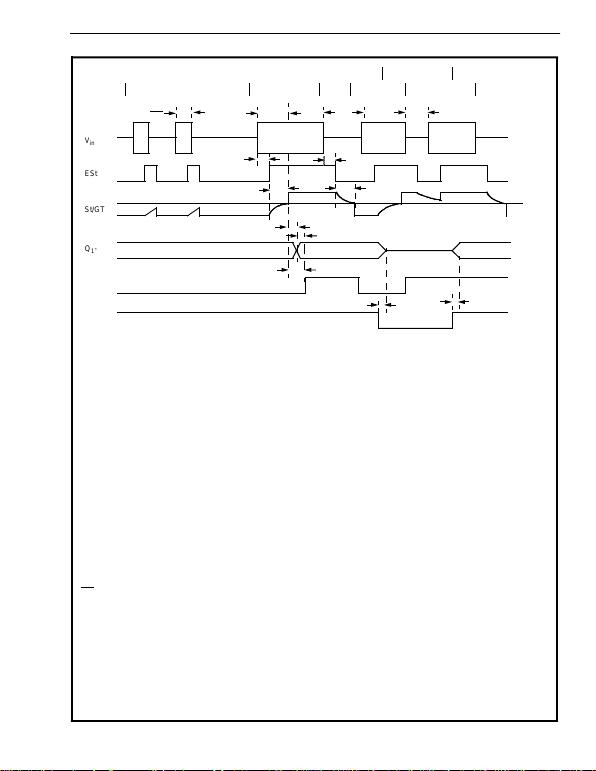ISO2-CMOS MT8870D/MT8870D-1
4-21
Figure 11 - Timing D iagram
AA
AA
AA
AA
AA
AA
AA
Vin
ESt
St/GT
Q1-Q4
StD
TOE
EVENTS ABC
D
EFG
t
REC tREC tID tDO
TONE #n TONE
#n + 1 TONE
#n + 1
tDP tDA
tGTP tGTA
tPQ tQStD
tPSrD
tPTD
tPTE
# n # (n + 1)
HIGH IMPEDAN CE
DECODED TONE # (n-1)
EXPLANATION OF EVENTS
EXPLANATION OF SYMBOLS
A) TONE BU RSTS DETECTED, TONE DURATION INVALID, OUTPUTS NOT UPDATED.
B) TONE #n DETECTED, TONE DURATION VALID, TONE DECODED AND LATCHED IN OUTPUTS
C) END OF TONE #n DETECTED, TONE ABSENT DURATION VALID, OUTPUTS REMIAN LATCHED UNTIL NEXT VALID
TONE.
D) OUTPUTS SWITCHED TO HIGH IMPEDANCE STATE.
E) TONE #n + 1 DETECTED, TONE DURATION VALID, TONE DECODED AND LATCHED IN OUTPUTS (CURRENTLY
HIG H IMPEDA N C E) .
F) ACCEPTABLE DROPOUT OF TONE #n + 1, TONE ABSENT DURATION INVALID, OUTPUTS REMAIN LATCHED.
G) END OF TONE #n + 1 DETECTED, TONE ABSENT DURATION VALID, OUTPUTS REMAIN LATCHED UNTIL NEXT
VALID TONE.
Vin DTMF COMPOSITE INPUT SIGNAL.
ESt EARLY STEERING OUTPUT. INDIC ATES DETECTION OF VALID TONE FREQUENCIES.
St/GT STEERING INPUT/GUARD TIME OUTPUT. DRIVES EXTERNAL RC TIMING CI RCUIT.
Q1-Q44-BIT DECODED TONE OUTPUT.
StD DELAYED STEERING OUTPUT. INDICATES THAT VALID FREQUENCIES HAVE BEEN PRESENT/ABSENT FOR THE
REQUIRED GUAR D TIM E THUS CONSTITUTING A VALID SIGNAL.
TOE TONE OUTPUT ENABLE (INPUT). A LOW LEVEL SHIFTS Q1-Q4 TO ITS HIGH IMPED ANCE S TATE.
tREC MAXIMUM DTM F SIGNAL DURATION NOT DETECE D AS VALID
tREC MINIMUM DTMF SIGNAL DURATIO N REQUIRED FOR VALID RECOGNITION
tID MAXIMUM TIME BETWEEN VALID DTMF SIGNALS.
tDO MA XI MUM A L LOWAB L E D R OP O U T D UR I NG VA L I D D TM F SI G N AL .
tDP TIME TO DETECT THE PRESENCE OF VALID DTM F SIGNALS.
tDA TIME TO DETECT THE ABSENCE OF VALID DTMF SIGNALS.
tGTP GUARD TIME, TONE PRESENT.
tGTA GUARD TIME, TONE ABSENT.
VTStMT8870D/MT8870D-1 ISO2-CMOS
4-22
NOTES: